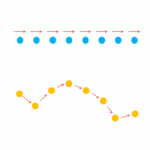Smartick is a fun way to learn math!# I want to learn about: Geometry

Learn elementary geometry with Smartick. Exercises, theory, tutorials, and teaching resources for children learning elementary geometry. Here you will find posts about geometry to make learning easier and more fun.

Aug04

## Distributive Property in Geometry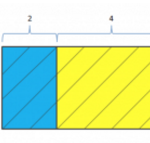In today’s post, we are going to take a look at the distributive property from a geometric point of view. You can consult these entries for more information on this property: Multiplication Properties Distributive Property from a Geometric point of view: We’re going to start by drawing a striped rectangle that’s divided into two smaller […]

Jun12

## Cylinder: Characteristics, Examples, and How to Calculate Their Area and Volume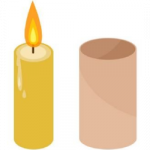Do you know what a cylinder is? In addition to explaining what cylinders are, in this post we will look at how they develop, the parts that make up a cylinder, and how to calculate its area and volume. We will also show you some activities using cylinders that children complete during their Smartick sessions. […]

May18

## The Relationship between Clocks and Angles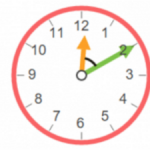In today’s post, we’re going to take a look at the relationship between clocks and angles. More specifically, we’ll see the kinds of angles that can be formed by the hands of a clock and how to calculate them. On a clock, we can see different kinds of angles based on its amplitude; void, acute, […]

May03

## Learn about Open and Closed Curves

Curved lines and straight lines. This week’s post will teach you how to differentiate between open and closed curves. The first item on our agenda is: Do you know what a curve is? A curve is a succession of points that constantly change direction, in other words, it isn’t straight. The image below shows a […]

Apr26

## Review the Properties of Polynomials

In this post we will look at the properties of polynomials, what they are, what degree they have, and whether or not they complete and/or in standard form. Let’s start by looking at what polynomials are. A polynomial is an assembly of monomials. Polynomials are usually named with capital letters (usually starting with the letter […]

Feb10

We are going to take a look at some straight lines in today’s post. The first thing that’s on the agenda is to define what exactly straight lines are in order to differentiate them from curved lines. Then, we’ll have a look at the concepts of rays, line segments, and open rays. What is a […]

Dec23

## Geometric Plane Shapes: Circles, Triangles, Rectangles, Squares, and Trapezoids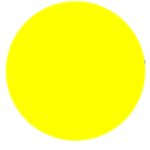What are geometric plane shapes? What characteristics do they have? These are the questions that we will answer in this post. The principal geometric plane shapes are: The Circle The circle is a shape that can be made by tracing a curve that is always the same distance from a point that we call the […]

Dec08

## Learn about Angles: Obtuse, Straight, Right and Acute AngleWhat’s an angle? Right, obtuse, straight, acute angle… what are these and what do they have to do with angles? If they can be measured… how can we measure them? What are they used for? …These are some of the questions that we’re going to answer in this post. So…let’s do this! Angles: An area marked […]

Oct18

## Angles Explained with Clockhands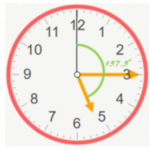In today’s post, we’ll be relating clocks to angles as we are learning how to calculate the angle that the two hands on a clock form. An analog clock has 12 numbers, which divide the clock into 12 equal parts. These divisions indicate the hours. Each hour is divided into five subdivisions that amount to […]